# Physics - Classical Mechanics - Explaining the Physics behind Rocket Propulsion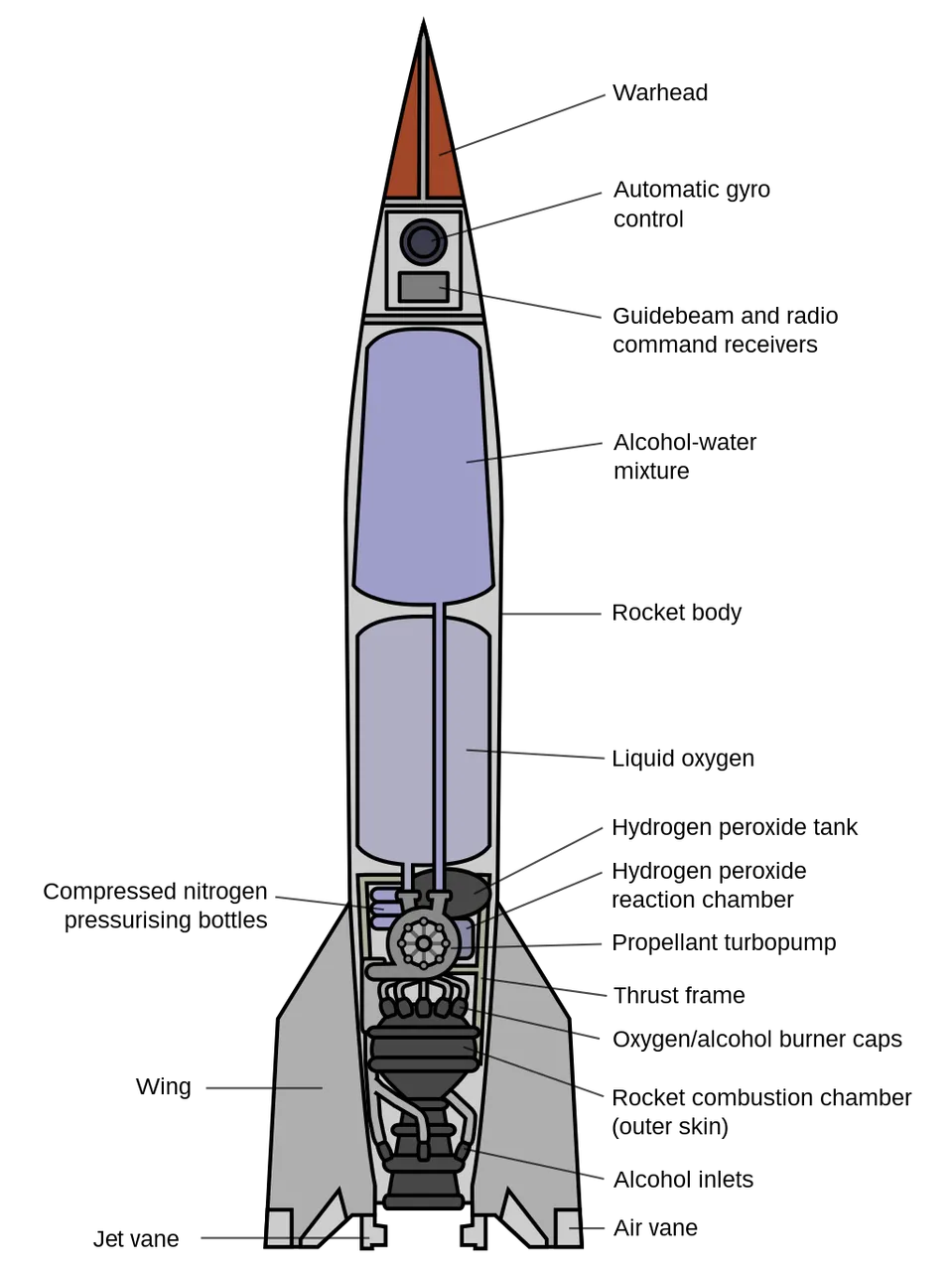[Image 1]

## Introduction

Hey it's a me again @drifter1! Today we continue with Physics and more specifically the branch "Classical Mechanics" to get into the Rocket Propulsion. So, without further ado, let's get straight into it!

## Required Physics Background

Important physics topics that you should understand before getting into Rocket Propulsion are all three of Newton's Laws of Motion and all the quantities that come with them (Momentum, Impulse etc.).

### Newton's First Law

The Law of Inertia tells us that objects tend to keep their current state of motion unless an unbalanced force acts upon them. So, an object at rest stays at rest, whilst an object with a constant velocity will continue moving in constant linear motion as long as the total sum of all forces that act on it is zero. This law is closely connected to the definition of Momentum (p = m•v), which is the quantity that tells us how much (mass) is moving and how fast (velocity) it's moving.

### Newton's Second Law

This law shows us how forces affect the motion of objects and how exactly the velocity and momentum change by the effect of forces. The basic definition of the law is F = m•a (Force equals mass times acceleration) and knowing that acceleration is simply the change in velocity (a = dv/dt) this can be changed into F = dp/dt, showing us that the force is equal to the change in momentum over time, a quantity which is known as Impulse J. Impulse is also equal to the area (integral) under a force-time curve. This law is sometimes also called the Impulse-Momentum Theorem.

### Newton's Third Law

This law is quite important as it states that for every action there is an opposite and equal re-action. Knowing that F1 = -F2, we can also talk about the conservation of momentum, telling us that the momentum of every system get's conserved.

### Combining the Laws

So, how does an rocket work? Well, an unbalanced force must be exerted for a rocket to lift it off from the launch pad or to change it's speed or direction in space (1st law). The amount of thrust (force) produced by a rocket engine is determined by the mass of the rocket fuel that is burned and how fast the gas escapes the rocket (second law). The reaction/motion of the rocket is equal and opposite to the direction of the action/thrust from the engine (third law).

## Rocket Propulsion Analysis

### Physics involved

Let's now get more in-depth to get mathematical equations that apply to rocket propulsion. In general, thinking about Newton's laws and all the other quantities that we can re-mentioned previously, we can describe the physics that are involved as:
• Momentum, caused by the continuously ejecting burned fuel gases, which have both mass and velocity. For momentum we can also consider:
• The conservation of momentum, which tells us that the rocket's momentum changes by the same amount/rate as the burning fuel gases(with opposite sign).
• The rate of change of momentum (impulse) is constant, assuming that the burned fuel is ejected at a constant rate.
• From Newton's second law, we can say that a constant force is applied on the rocket.
• As time goes by, the mass of the rocket (including the remaining fuel) continuously decreases. Defining that the force is constant, from Newton's second law we can say that the acceleration is constantly increasing, as the mass is decreasing.
• The total change of the rocket's velocity therefore depends on the amount of mass of fuel that is burned. This dependence is not linear.
So, in the end, the problem of rocket propulsion is about the mass and velocity of the rocket and ejected gases that are changing. Defining the system to be rocket + fuel, which is clearly a closed system (for deep space where there are no external forces acting on it), we can say that the momentum is conserved for this system. So, we can apply the conservation of momentum.

### The rocket + fuel system

The rocket + fuel system can be visualized like this: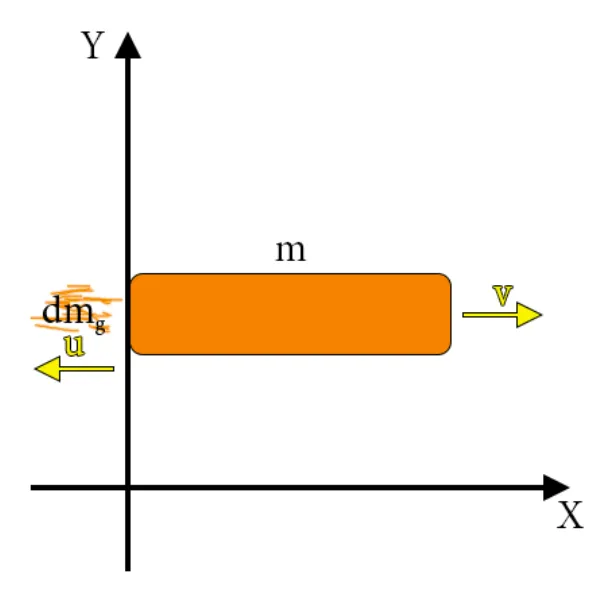[Based on Figure 9.7.2 from phys.libretexts.org]

Where:
• m is the total instantaneous rocket mass (mass of the rocket body + the mass of the remaining fuel at that point of time)
• v is the rocket's instantaneous velocity, which is measured relative to an inertial reference system (like the Earth). So, the initial momentum of the system is p=m•v.
• u is the velocity or rate at which infinitesimal masses of gas dmg are ejected from the engine in the opposite direction. This velocity is measured in respected to the moving rocket and not the Earth. In respect to the Earth the exhaust gas has a velocity of (v - u)

### Mathematical Analysis

Because of the ejection of fuel mass the rocket's mass decreases by dmg and its velocity increase by dv. Including both the change for the rocket and the exhaust gas, the final momentum of the system is: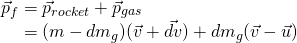Considering that the motion takes place in one axis (x-axis) and that the vectors are all in the x-direction, we can drop the vector notation. Applying the conservation of momentum we obtain:The quantities dmg and dv are each very small, therefore their product dmg•dv is also very, very small...much smaller than the other two terms in the expression. So, we can easily neglect this term and obtain: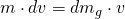The quantity dmg represents the increase in the mass of ejected gases, which means that it also represents the decrease of mass of the rocket (-dm). So, replacing it in the previous equation we get: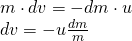Last but not least, we have to integrate from the initial mass mi to the final mass m of the rocket (and initial velocity vi to the final velocity v), which gives us the change in velocity:This final result is called the rocket equation, and was originally derived by the Soviet physicist Konstantin Tsiolkovsky in 1897. This equation gives us the change of velocity that the rocket obtains from burning a mass of fuel which decreases the total rocket mass from mi down to m. The relationship between Δv and the change of mass of the rocket is clearly non-linear, as we mentioned earlier in this article.

### Rocket in Gravitational Field

Being in a Gravitational Field, the rocket's velocity is affected by the constant acceleration of gravity g. Doing similar calculations we end up with a very similar equation that looks like this: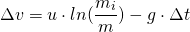In this equation, Δt is the burn time of fuel. In absence of gravity, there is no difference on how much time it takes to burn the entire mass of fuel, as the change of velocity didn't depend on Δt. But, in presence of gravity, it matters a lot. So, the longer the burn time, the smaller the rocket's change of velocity will be. That's exactly why rockets launch off in a spectacular at the first moment liftoff. It's essential to burn the fuel as quickly as possible, to get the largest Δv that is possible.

## Takeaways

So, what have you learned today?
1. Rocket propulsion is quite complex, but based on Newton's motion laws and quantities closely connected to them (momentum, impulse etc.)
2. The closed system of a rocket contains both the rocket's body mass and the fuel mass (rocket + fuel system)
3. The change of velocity of the rocket is closely connected to the rate at which we lower the rocket's mass by burning/ejecting fuel mass.
4. The greater the exhaust velocity u, the faster the rocket burns its fuel and the smaller the rocket's mass is (all are referring to a decrease of mass), the greater the acceleration of the rocket will be.
5. When in a gravity field, the total change in velocity is greater when the fuel burn time is small. In Deep space, on the other hand, the change of velocity does not depend on the burn time.

## RESOURCES:

### References

1. https://www.grc.nasa.gov/www/k-12/rocket/TRCRocket/rocket_principles.html
2. https://phys.libretexts.org/Bookshelves/University_Physics/Book%3A_University_Physics_(OpenStax)/Map%3A_University_Physics_I_-_Mechanics%2C_Sound%2C_Oscillations%2C_and_Waves_(OpenStax)/9%3A_Linear_Momentum_and_Collisions/9.7%3A_Rocket_Propulsion
3. https://opentextbc.ca/physicstestbook2/chapter/introduction-to-rocket-propulsion/
4. http://hyperphysics.phy-astr.gsu.edu/hbase/rocket.html#c4

### Images

1.## Final words | Next up

This is actually it for today's post! Next time we will start getting into the rotation of objects, starting off with angular velocity and acceleration...

See ya!Keep on drifting!

H2
H3
H4
3 columns
2 columns
1 column